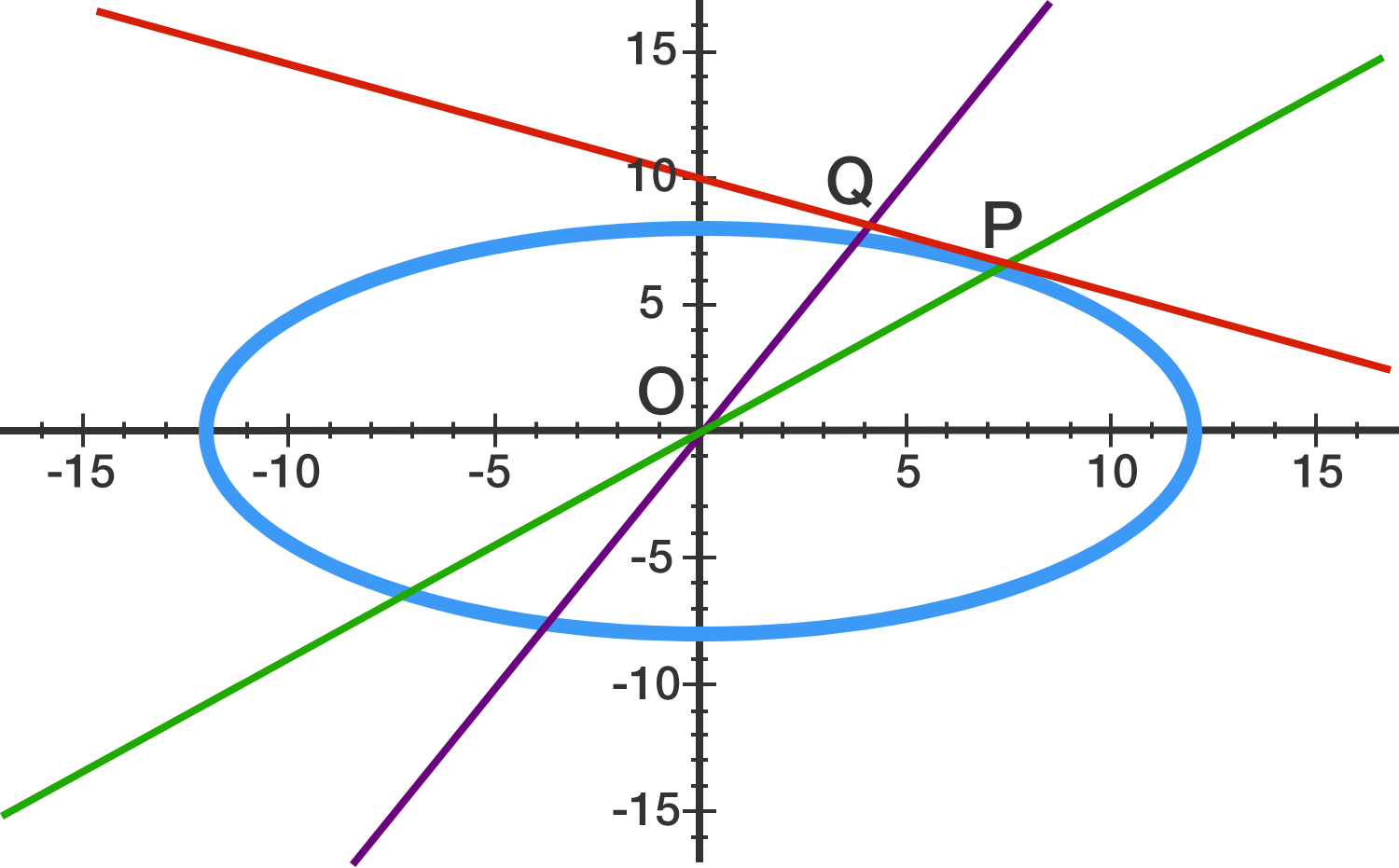# Maximum area of a strange triangle

Geometry Level 3Consider an ellipse $\dfrac{x^2}{144} + \dfrac{y^2}{64} = 1$. A line is drawn tangent to the ellipse at a point $P$. A line segment drawn from the origin to a point $Q$ on this line is perpendicular to this tangent line.

Find the maximum area of $\triangle POQ$.

×